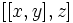# Proof of generalized Baer construction of Lie ring for class two 2-group with a suitable cocycle

Jump to: navigation, search

This proof is instrumental in the definitions of generalized Baer IIP Lie ring and generalized Baer cyclicity-preserving Lie ring.

## Statement

Suppose$P$ is a class two group. Suppose$A$ is a central subgroup of$P$ and$G \cong A/P$ is an abelian group (in particular, this means that$[P,P] \le A \le Z(P)$.$f$ is a function$f:P \times P \to A$ such that$f$ is constant in each input on the cosets of$A$. Denote by$\overline{f}$ the induced function$P/A\times P/A \to A$. Suppose the following four conditions are satisfied:

1. The cocycle condition: This states that$f(x,y)f(xy,z) = f(x,yz)f(y,z)$ for all$x,y,z \in P$. This is equivalent to requiring that$\overline{f}$ be a 2-cocycle from$P/A$ to$A$.
2. The skew equals commutator condition: This states that$f(x,y)(f(y,x))^{-1} = [x,y]$.
3. The identity-preservation condition: This states that$f(x,e) = f(e,x) = e$ for all$x$, where$e$ is the identity element.
4. The inverse-preservation condition: This states that$f(x,x^{-1}) = e$ for all$x$, where$e$ is the identity element.

We give$P$ the structure of a Lie ring as follows:

• The addition is given by:$x + y := \frac{xy}{f(x,y)}$

• The additive identity for the Lie ring is the group's identity element.
• Additive inverses are the same as multiplicative inverses in the group.
• The Lie bracket is the same as the commutator in the group.

The claim is that with these operations,$P$ acquires the structure of a class two Lie ring.

### Additional claims

1. We say that$f$ is cyclicity-preserving if, whenever$x,y \in P$ satisfy the property that$\langle x,y \rangle$ is cyclic, then$f(x,y) = e$. If$f$ is cyclicity-preserving, the identification between$P$ and the additive group of its generalized Baer Lie ring for$f$ gives a 1-isomorphism of groups. In particular,$P$ is 1-isomorphic to an abelian group.

### Note

Note that the statement and construction work for odd-order$p$-groups as well, but in those cases,$f$ is uniquely determined and is denoted as$\sqrt{[x,y]}$. This is the Baer correspondence. For full proof, refer: Proof of Baer construction of Lie ring for odd-order class two p-group

The proof can be given using an interpretation in terms of twisting the cohomology class of the extension. PLACEHOLDER FOR INFORMATION TO BE FILLED IN: [SHOW MORE]

## Proof (cohomology interpretation)

PLACEHOLDER FOR INFORMATION TO BE FILLED IN: [SHOW MORE]

## Proof (hands-on)

### Comment on the operation being well-defined

Since$f(x,y)$ is central, we do not need to specify, when dividing by it, whether we are dividing on the left or the right. Thus, the fraction notation is unambiguous.

### Addition is associative

To prove:$\! (x + y) + z = x + (y + z)$

Key proof ingredient: The cocycle condition.

Proof: [SHOW MORE]

### Addition is commutative

To prove:$\! x + y = y + x$

Key proof ingredient: Skew is commutator.

Proof: [SHOW MORE]

### Agreement of identity and inverses

To prove: If$e$ is the identity element for the multiplication, then$x + e = e + x = x$ and$x + x^{-1} = x^{-1} + x = e$.

Key proof ingredient: Identity-preservation and inverse-preservation.

Proof: [SHOW MORE]

### The Lie bracket is additive in the first variable

To prove:$\! [x + y,z] = [x,z] + [y,z]$

Proof: [SHOW MORE]

### The Lie bracket is additive in the second variable

This is analogous to additivity in the first variable, as shown above.

### The Lie bracket is alternating

This follows from the fact that the commutator of an element with itself is the identity.

### The Jacobi identity and class two

Since the Lie bracket coincides with the commutator, and the commutator satisfies that$[[x,y],z]$ is trivial for all$x,y,z$, the Lie bracket also satisfies the same condition. Thus, it satisfies Jacobi's identity and also the condition for class two.